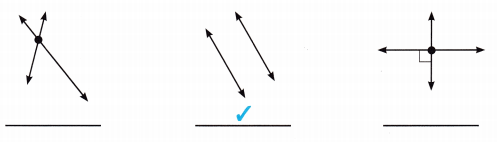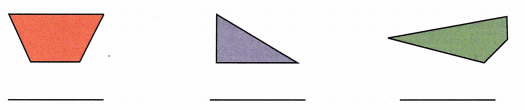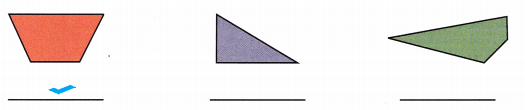# McGraw Hill Math Grade 4 Chapter 12 Lesson 4 Answer Key Parallel and Perpendicular Lines

Practice the questions of McGraw Hill Math Grade 4 Answer Key PDF Chapter 12 Lesson 4 Parallel and Perpendicular Lines to secure good marks & knowledge in the exams.

## McGraw-Hill Math Grade 4 Answer Key Chapter 12 Lesson 4 Parallel and Perpendicular Lines

Solve

Question 1.
Which pair of lines is parallel? Place a checkmark under the correct answer.Explanation:
The second pair of lines are parallel lines
So, i placed a check mark under the second pair of lines.

Question 2.
Which shape shows perpendicular lines?Explanation:
The third shape shows the perpendicular lines
So, i placed a check mark under the third shape.

Question 3.
Which shape shows parallel lines?